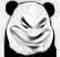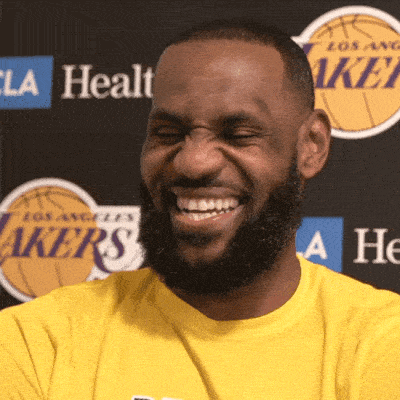NBA英雄

NBA范特西

# 【字幕】贝弗利谈詹库历史地位：如果勇士再拿一冠，FMVP库里能和詹姆斯平起平坐

1859回复/ 50446598 浏览【字幕】贝弗利谈詹库历史地位：如果勇士再拿一冠，FMVP库里能和詹姆斯平起平坐文森篮球 发表在湿乎乎的话题 https://bbs.hupu.com/vote

[图片]……

……[图片][图片][图片][图片]4+4+4=2+4+1？我不理解

4+4+4=2+4+1？我不理解

[图片][图片]🐶哥你变了，甚至承认我库长得帅🐶哥你变了，甚至承认我库长得帅[图片][图片]4+4+4=2+4+1？我不理解

4+4+4=2+4+1？我不理解[图片]id好评 我可太想这两人一个队了

id好评 我可太想这两人一个队了

4+4+4=2+4+1？我不理解

4+4+4=2+4+1？我不理解[图片]Re：【字幕】贝弗利谈詹库历史地位：如果勇士再拿一冠，FMVP库里能和詹姆斯平起平坐